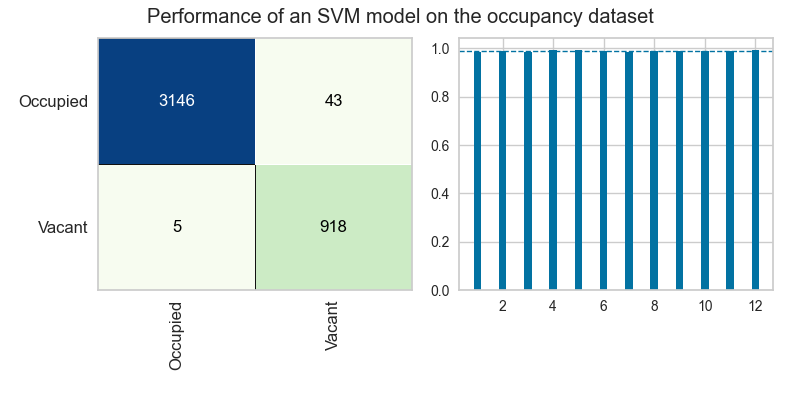# Subplots with Yellowbrick

## June 08, 2020

Reading time ~1 minute

In this quick post, we’ll see how to use Yellowbrick together with Matplotlib’s subplots feature to create a visual story about the modeling process:# Creating the subplots

First we have to use Matplotlib’s subplots feature to create a figure that will be able to contain two side-by-side subplots:

``````fig, axes = plt.subplots(ncols=2)
``````

This will create a `fig` object, which is the entire space of our plot, as well as an `axes` object, which contains two `ax` objects that we will be able to access separately with numerical indexing.

Note: If we had written `plt.subplots(nrows=2, ...)` we’d get two plots stacked on top of each other instead of side-by-side.

# Confusion Matrix

The Yellowbrick `ConfusionMatrix` visualizer is designed to help us understand where our model is making mistakes in classifying the training data. Because the visualizer accepts an `ax` parameter, we can pass in the coordinates of our first `ax` object directly into the visualizer on instantiation to tell it to position itself inside those coordinates on the figure we’ve created above.

``````confusion_matrix = ConfusionMatrix(
model,
ax=axes
)
``````

# Cross-Validation

The Yellowbrick `CVScores` visualizer is part of the `model_selection` module, and is aimed at helping us to validate that our model is performing about equally well on all slices of our data during cross-validation. The `CVScores` visualizer also accepts an `ax` parameter:

``````cv_scores = CVScores(
model,
ax=axes,
scoring="f1_weighted",
cv=StratifiedKFold(n_splits=12, shuffle=True)
)
``````

This code tells Yellowbrick to put the `CVScores` plot into the second of the two axes we created.

# Putting all the pieces together

Here are all the pieces, put together (with a few other stylistic additions):

Note that in the above, we also use Matplotlib’s `tight_layout` method to encourage all of the features of the subplots to fit together nicely inside the figure. It’s very helpful when using many subplots! Check out this post for more examples of how to use Matplotlib effectively!

### A Parrot Trainer Eats Crow

In this post, we'll consider how it is that models trained on massive datasets using millions of parameters can be both "low bias" and al...… Continue reading

#### Why don't developers give much cred to low-code/no-code tools?

Published on February 24, 2021

#### Embedded Binaries for Go

Published on February 06, 2021